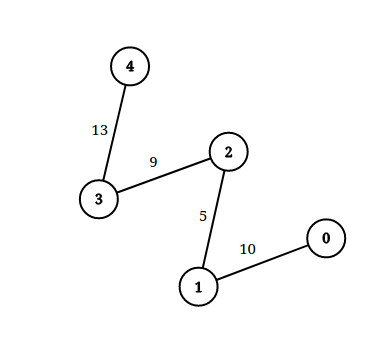1697. Checking Existence of Edge Length Limited Paths

An undirected graph of `n` nodes is defined by `edgeList`, where `edgeList[i] = [ui, vi, disi]` denotes an edge between nodes `ui` and `vi` with distance `disi`. Note that there may be multiple edges between two nodes.

Given an array `queries`, where `queries[j] = [pj, qj, limitj]`, your task is to determine for each `queries[j]` whether there is a path between `pj` and `qj` such that each edge on the path has a distance strictly less than `limitj` .

Return a boolean array `answer`, where `answer.length == queries.length` and the `jth` value of `answer` is `true` if there is a path for `queries[j]` is `true`, and `false` otherwise.

Example 1:```Input: n = 3, edgeList = [[0,1,2],[1,2,4],[2,0,8],[1,0,16]], queries = [[0,1,2],[0,2,5]]
Output: [false,true]
Explanation: The above figure shows the given graph. Note that there are two overlapping edges between 0 and 1 with distances 2 and 16.
For the first query, between 0 and 1 there is no path where each distance is less than 2, thus we return false for this query.
For the second query, there is a path (0 -> 1 -> 2) of two edges with distances less than 5, thus we return true for this query.
```

Example 2:```Input: n = 5, edgeList = [[0,1,10],[1,2,5],[2,3,9],[3,4,13]], queries = [[0,4,14],[1,4,13]]
Output: [true,false]
Exaplanation: The above figure shows the given graph.
```

Constraints:

• `2 <= n <= 105`
• `1 <= edgeList.length, queries.length <= 105`
• `edgeList[i].length == 3`
• `queries[j].length == 3`
• `0 <= ui, vi, pj, qj <= n - 1`
• `ui != vi`
• `pj != qj`
• `1 <= disi, limitj <= 109`
• There may be multiple edges between two nodes.

1697. Checking Existence of Edge Length Limited Paths
``````struct Solution;

struct UnionFind {
parent: Vec<usize>,
n: usize,
}

impl UnionFind {
fn new(n: usize) -> Self {
let parent = (0..n).collect();
UnionFind { parent, n }
}

fn find(&mut self, i: usize) -> usize {
let j = self.parent[i];
if j != i {
let k = self.find(j);
self.parent[i] = k;
k
} else {
i
}
}

fn union(&mut self, i: usize, j: usize) {
let i = self.find(i);
let j = self.find(j);
if i != j {
self.parent[i] = j;
}
}
}

impl Solution {
fn distance_limited_paths_exist(
n: i32,
edge_list: Vec<Vec<i32>>,
queries: Vec<Vec<i32>>,
) -> Vec<bool> {
let n = n as usize;
let mut edges: Vec<(i32, usize, usize)> = edge_list
.into_iter()
.map(|e| (e, e as usize, e as usize))
.collect();
edges.sort_unstable();
edges.reverse();
let mut queries: Vec<(i32, usize, usize, usize)> = queries
.into_iter()
.enumerate()
.map(|(i, q)| (q, q as usize, q as usize, i))
.collect();
queries.sort_unstable();
let m = queries.len();
let mut res = vec![false; m];
let mut uf = UnionFind::new(n);
for (qd, qi, qj, qid) in queries {
while let Some(&(ed, ei, ej)) = edges.last() {
if ed < qd {
edges.pop();
uf.union(ei, ej);
} else {
break;
}
}
if uf.find(qi) == uf.find(qj) {
res[qid] = true;
}
}
res
}
}

#[test]
fn test() {
let n = 3;
let edge_list = vec_vec_i32![[0, 1, 2], [1, 2, 4], [2, 0, 8], [1, 0, 16]];
let queries = vec_vec_i32![[0, 1, 2], [0, 2, 5]];
let res = vec![false, true];
assert_eq!(
Solution::distance_limited_paths_exist(n, edge_list, queries),
res
);
let n = 5;
let edge_list = vec_vec_i32![[0, 1, 10], [1, 2, 5], [2, 3, 9], [3, 4, 13]];
let queries = vec_vec_i32![[0, 4, 14], [1, 4, 13]];
let res = vec![true, false];
assert_eq!(
Solution::distance_limited_paths_exist(n, edge_list, queries),
res
);
}
``````